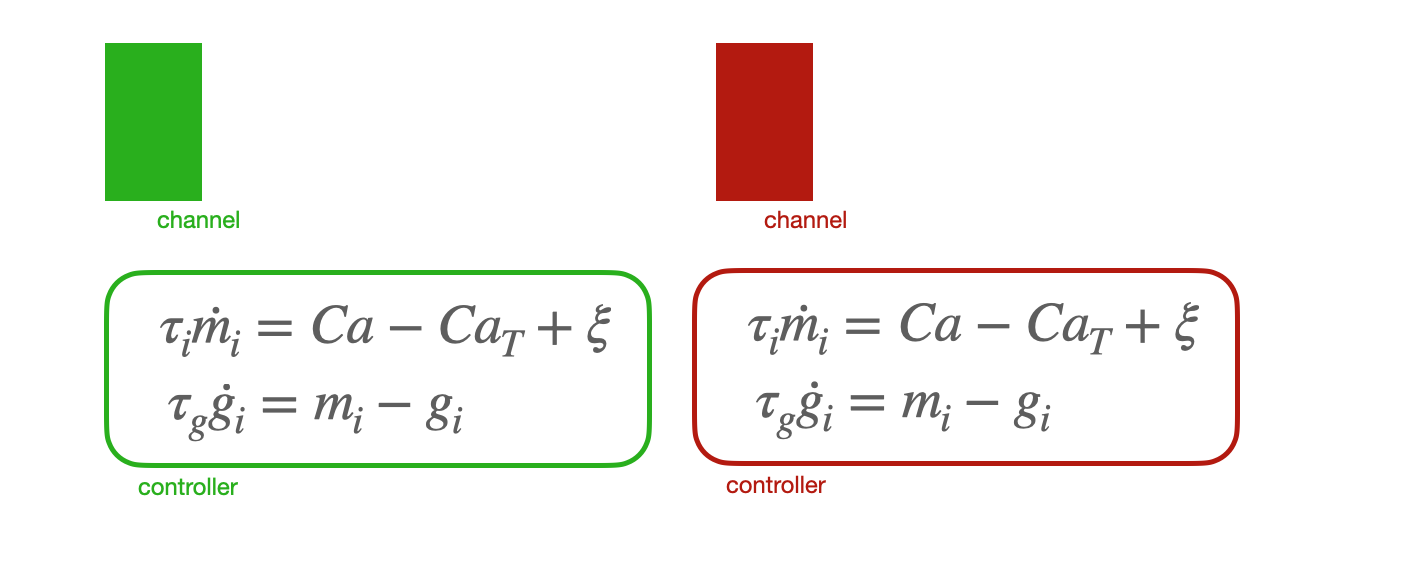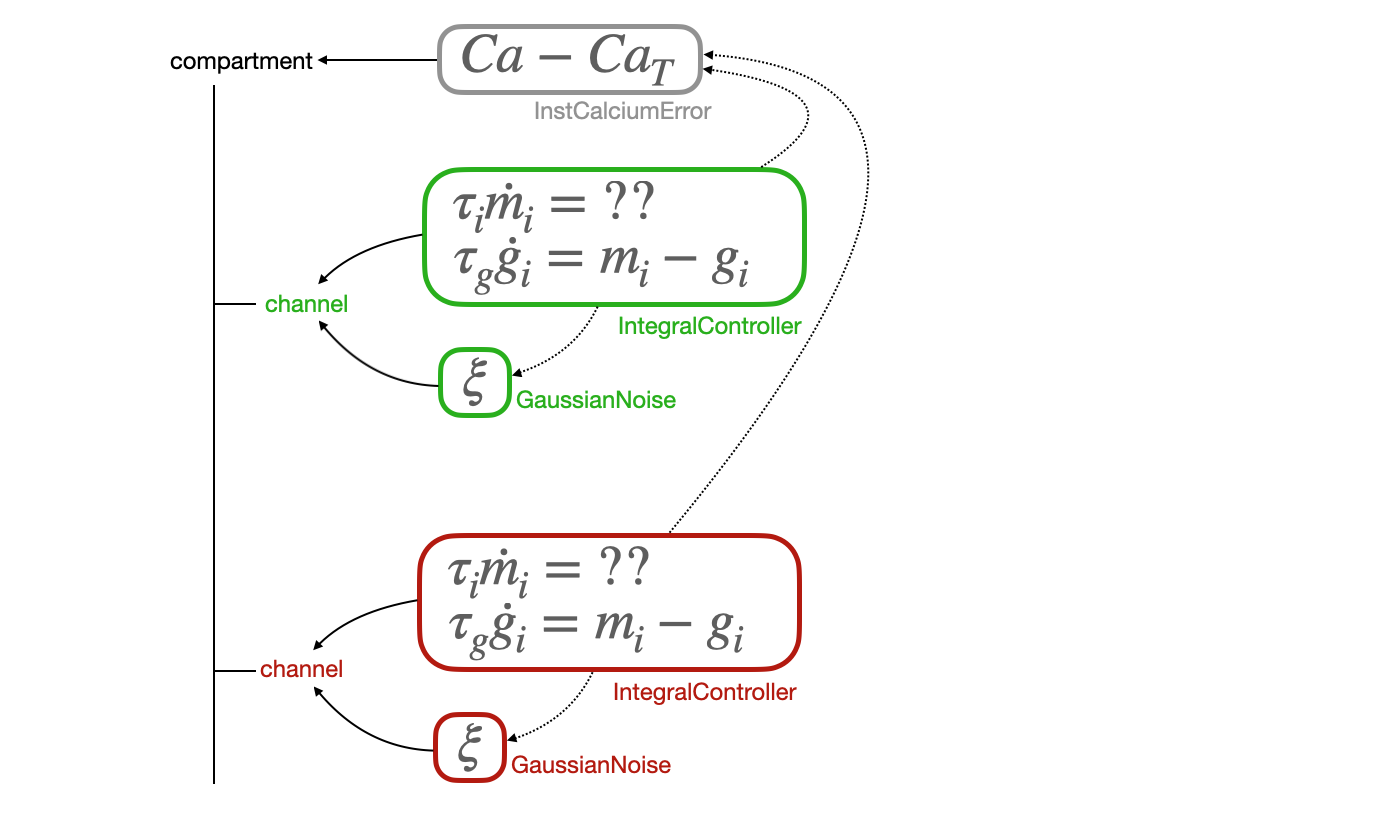# Construct complex self-assembling models

In this document, we will go over the process of implementing mechanisms that simulate a new model. This new model:

• changes the conductance of one of the channels dynamically
• based on the calcium levels in the cell
• and can be affected by noise

The way we implement the model will be implement three different mechanisms, instead of one monolithic piece of code. This has the following advantages:

1. individual mechanisms correspond to conceptually distinct things.
2. Mixing and matching mechansisms becomes easier, allowing us to extend this more easily.
3. This architecture is particuraly suited to ODEs with mulitple additive terms, where each term is its own mechanism.

## An example model¶

We will simulate a regulation model, based on O'Leary et al.

This is the core set of ODEs we are trying to model:

This regulation model applies to each channel i in the neuron, so we would imagine whatever mechanism we create to be added to each channel.

## Monolithic architecture¶Let's imagine what happens if we take these ODEs and stick them all into a single mechanism. We have some problems:

• The (Ca- Ca_T) term really belongs to the compartment, not individual channels, because it is true for all channels
• This means that we have to manually specify that it's the same for all channels, and also gives us the freedom to allow them to be different (which is bad, because this is not in our model)
• If we wanted to use the noise term in a completely different model, we really can't
• If we wanted to replace the first two terms in the first ODE with something else, there's no easy way to do it.

In summary, this monolithic architecture is brittle, and doesn't allow for expansion and limits our flexibility in the model.

## Self-assembling architecture¶An alternate way of writing this model is to use a "self-assembling" architecture, where we break up the model into the smallest conceptual pieces, and make each piece its own mechanism. Here, we have the following mechanisms:

1. InstCalciumError, which belongs to a compartment, and measures the instantenous difference between the calcium in that compartment and some target.
2. IntegralController, which changes implements the ODEs we discussed initially, but allows us to pass whatever we want into the RHS of the first ODE
3. GaussianNoise, which generates some Gaussian random noise.

We would create a model that looked like this using:

% assuming x is a xolotl model with a compartment named C

% and so on for the other channels


OK, this looks good, but how do these mechanisms know how to connect to each other (the dotted lines in the diagram). For this model to work correctly,

• IntegralController needs to connect to InstCalciumError so it can read the first term of the RHS of the m ODE
• IntegralController needs to connect to the correct GaussianNoise mechanism (the one that is connected to the same channel it is) to read out the second term of the RHS of the m ODE.

xolotl provides several methods to automatically allow mechanisms to self-assemble. Let's review some of them.

## Methods to self-assemble inter-connected mechanisms¶

### findMechanismsOfType¶

How do we make sure the IntegralController mechanism connects to the InstCalciumError in the compartment? Because InstCalciumError is the only one of that type in the compartment, we can simply ask it to find mechanisms of that type and connect to that. In the init method, we can do something like this:

void IntegralController::init() {
RHS_terms = findMechanismsOfType("calcium_error");


where RHS_terms is of type vector<mechanism*>. Now this mechanism has a list of pointers to all mechanisms of this type in the compartment. This allows us to easily extend this model to add another additive term to the ODE -- simply add a new a new mechanism and declare it of type "calcium_error" and it will be automatically wired up.

Where (and how) does one declare mechanism types? Typically this is done in the constructor. For example, this is how we identify InstCalciumError as a mechanism of type "calcium_error"

// constructor for InstCalciumError
InstCalciumError(...) {
name = "InstCalciumError";
mechanism_type = "calcium_error";
}


### findMechanismsControlling¶

How do we make sure the IntegralController mechanism connects to the GaussianNoise that is connected to the same channel as it? This problem is tricky because there are many GaussianNoise mechanisms in the same compartment, and we need to connect to one and only the one that is also connected to the same channel. That's where this method comes in, which returns a vector of pointers to mechanisms that control the same object as the mechanism we are in.

void IntegralController::init() {
RHS_terms = findMechanismsOfType("calcium_error");

vector <mechanism*> A = findMechanismsControlling(this->controlling_class.c_str())

RHS_terms.insert(RHS_terms.end(), A.begin(), A.end());


You don't have to manually set controlling_class -- this is done automatically on model creation.